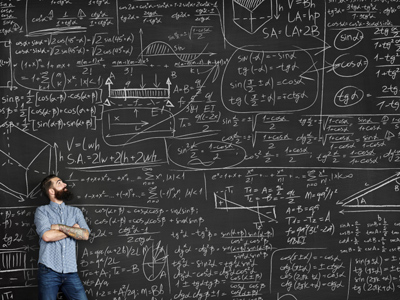There are three particularly challenging questions in this quiz.

# Number Sequences (Difficult)

Number sequences involve rules and finding them can be a bit of a challenge at times! This is our fourth and final section of 11-plus Maths quizzes on number sequences. If you haven't played the other three yet, it might be a good idea to go back and do them first. Some of these questions are particularly tricky, so take your time and think carefully before choosing your answer.

You may think that number sequences are not an important part of Maths. The thing with numbers is, the more you are familiar with how they work, the better you'll be in whichever career you choose. Plenty of careers require a solid knowledge of numbers, such as any of the sciences, and if you want a good career then learn your numbers!

Time for the quiz. You may find a pencil & paper handy for these questions. Good luck.

1.
Find the missing term.
39, 52, 65, X, ...
79
77
78
81
The next term is got from the previous term by adding 13, e.g. 39 + 13 = 52 and so on
2.
Find the missing term.
79, 95, X, 127, ...
111
110
103
115
The next term is got from the previous term by adding 16, e.g. 79 + 16 = 95 and so on
3.
Find the missing term.
-17, -11, -5, X, ...
1
0
-1
2
The next term is got from the previous term by adding 6, e.g. -17 + 6 = -11 and so on
4.
Find the missing term.
-10, -7, -4, X, ...
2
0
1
-1
The next term is got from the previous term by adding 3, e.g. -7 + 3 = -4 and so on
5.
Find the missing term.
1, 1, 2, 3, 5, 8, X, ...
11
13
12
10
This is the famous Fibonacci sequence. Each term is formed by the sum of the previous TWO terms: 1 + 1 = 2, 1 + 2 = 3, 2 + 3 = 5, 3 + 5 = 8, 5 + 8 = 13 and so on
6.
Find the missing term.
1, 8, 27, X, ...
81
49
36
64
The terms of this sequence are the counting numbers cubed: 13 = 1; 23 = 8; 33 = 27; 43 = 64; 53 = 125 and so on
7.
Find the missing term.
-4, 3, 10, X, ...
17
16
15
14
The next term is got from the previous term by adding 7, e.g. -4 + 7 = 3 and so on
8.
Find the missing term.
10, 100, 1,000, X, ...
10,000,000
10,000
100,000
1,000,000
The next term is got from the previous term by multiplying by 10, e.g. 10 × 10 = 100 and so on
9.
Find the missing term.
0, 4, 18, 48, X, 180, ...
96
120
100
90
The rule for the nth term = n3 - n2. Put in the values of 1, 2, 3, 4, ... in turn and see for yourself how the sequence is produced
10.
Find the missing term.
3, 7, 11, X, ...
14
16
15
17
The next term is got from the previous term by adding 4, e.g. 3 + 4 = 7 and so on
Author:  Frank Evans